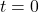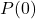## A species of animal is discovered on an island. Suppose that the population size of the species can be modeled by the following function, wh

Question

A species of animal is discovered on an island. Suppose that the population size of the species can be modeled by the following function, where time t is measured in years. P(t) = 520/1 + 8e-0.3t Find the initial population size of the species and the population size after years.

in progress 0
5 months 2021-08-13T17:34:53+00:00 1 Answers 56 views 0

## Answers ( )

The initial population size is of 58.

The population size of the specie after t years is given by:

*** QuickLaTeX cannot compile formula:
P(t) = \frac{520}{1 + 8e^{-0.3t}

*** Error message:
File ended while scanning use of \frac .
Emergency stop.



Step-by-step explanation:

Population size of the specie:

The population size of the specie after t years is given by:

*** QuickLaTeX cannot compile formula:
P(t) = \frac{520}{1 + 8e^{-0.3t}

*** Error message:
File ended while scanning use of \frac .
Emergency stop.



Initial population size

This is P when, that is,. So

*** QuickLaTeX cannot compile formula:
P(0) = \frac{520}{1 + 8e^{-0.3*0} = \frac{520}{1+8} = \frac{520}{9} = 57.7

*** Error message:
File ended while scanning use of \frac .
Emergency stop.



Rounding to the nearest number, 58

The initial population size is of 58.